2.4. String loops and scaling

We saw before the reasons why gauge monopoles and domain walls were a bit of a problem for cosmology. Essentially, the problem was that their energy density decreases more slowly than the critical density with the expansion of the universe. This fact resulted in their contribution todef (the density in defects normalized by the critical density) being largely in excess compared to 1, hence in blatant conflict with modern observations. The question now arises as to whether the same might happened with cosmic strings. Are strings dominating the energy density of the universe? Fortunately, the answer to this question is no; strings evolve in such a way to make their densitystrings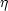2 t-2. Hence, one gets the same temporal behavior as for the critical density. The result is thatstrings ~ Gµ ~ (/ mP)2 ~ 10-6 for GUT strings, i.e., we get an interestingly small enough, constant fraction of the critical density of the universe and strings never upset standard observational cosmology.

Now, why this is so? The answer is simply the efficient way in which a network of strings looses energy. The evolution of the string network is highly nontrivial and loops are continuously chopped off from the main infinite strings as the result of (self) intersections within the infinite-string network. Once they are produced, loops oscillate due to their huge tension and slowly decay by emitting gravitational radiation. Thus, energy is transferred from the cosmic string network to radiation. (11)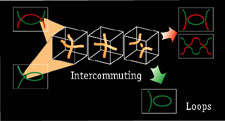Figure 5. Global string interactions leading to loop formation. Whenever two string segments intersect, they reconnect or intercommute (green and red strings - upper part of the figure). Analogously, if a string intersects itself, it can break off a closed loop (green string - bottom part of the figure). In both cases, the interacting string segments first suffer a slight deformation (due to the long-range forces present for global strings), they subsequently fuse and finally exchange partners. A ephemeral unstable amount of energy in the form of a small loop remains in the middle where the energy is high enough to place the Higgs field in the false vacuum. It then quickly collapses, radiating away its energy. The situation is roughly the same for local strings, as simulations have shown.

It turns out from simulations that most of the energy in the string network (roughly a 80%) is in the form of infinite strings. Soon after formation one would expect long strings to have the form of random-walk with characteristic step given by the correlation length. Also, the typical distance between long string segments should also be of order. Monte Carlo simulations show that these strings are Brownian on sufficiently large scales, which means that the lengthof a string is related to the end-to-end distance d of two given points along the string (with d >>) in the form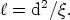(8)

What remains of the energy is given in the form of closed loops with no preferred length scale (a scale invariant distribution) which implies that the number density of loops having sizes between R and R + dR follows just from dimensional analysis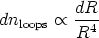(9)

which is just another way of saying that nloops1/R3, loops behave like normal nonrelativistic matter. The actual coefficient, as usual, comes from string simulations.

If we take(t) to be the mean number of infinite string segments per Hubble volume, then the energy density in infinite stringsstrings =s is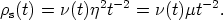(10)

Now,strings will typically haveintersections, and so the number of loops nloops(t) = nl(t) produced per unit volume will be proportional to2. We find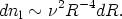(11)

Hence, recalling now that the loop sizes grow with the expansion like Rt we have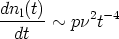(12)

where p is the probability of loop formation per intersection, a quantity related to the intercommuting probability, both roughly of order 1. We are now in a position to write an energy conservation equation for strings plus loops in the expanding universe. Here it is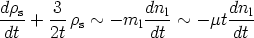(13)

where ml = µt is just the loop mass and where the second on the left hand side is the dilution term 3 Hs for an expanding radiation-dominated universe. The term on the right hand side amounts to the loss of energy from the long string network by the generation of small closed loops. Plugging Eqs. (10) and (12) into (13) Vilenkin finds the following kinetic equation for(t)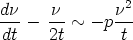(14)

with p ~ 1. Thus if>> 1 then d/ dt < 0 andtends to decrease in time, while if<< 1 then d/ dt > 0 andincreases. Hence, there will be a stable solution with~ a few.

11 High-resolution cosmic string simulations can be found in the Cambridge cosmology page at http://www.damtp.cam.ac.uk/user/gr/public/cs_evol.html Back.